## COUNTING CARS [DAY 2]

Explore addition through the strategy of counting on and the part whole model.

## Intentionality & Unit Overview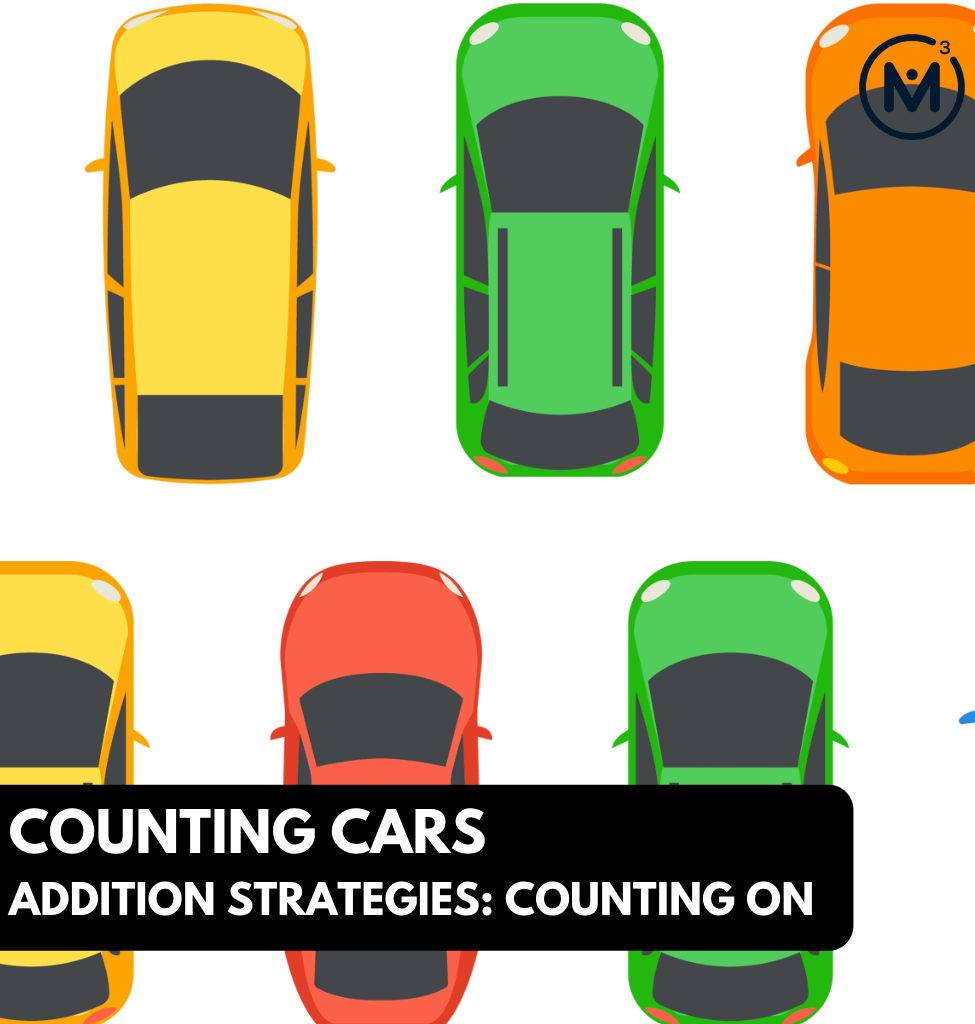### Access each lesson from this unit using the navigation links below

Students will explore number strings and purposeful practice related to addition. Each will serve to develop a deeper understanding of the strategy of counting on. The idea of near numbers should be established in preparation of these number talks.

The purpose of the Day 2 activities is to reinforce key concepts from Day 1. Students will engage in visual number strings to encourage the action associated with counting on (storing one number in our head and tracking the second amount). The students will have an opportunity to practice this strategy with a game and in a small group activity.

Big ideas of the unit:

• Addition names the whole in terms of the parts: when the parts of a set are known we call it addition
• The part whole relationship is connected to addition as numbers are composed of two or more parts
• Addition can be used in action (or active) situations (i.e.: joining and separating) and static (or passive) situations (i.e.: part part whole)
• Different addition situations will elicit different strategies
• Number relationships provide the foundation for strategies to help students remember basic facts
• Using the part whole model to understand the unknown parts of a problem. The unknown identified which operation needs to be used to solve the problem
• Provide students an opportunity to visualize a situation then interpret which operation is needed to  solve word problems
• Models can be used to connect concrete to abstract
• Explore addition strategies and provide opportunities for students to move from “direct modelling and counting all” to using counting on as a strategy to solve an addition question. Counting on is an important development as students work with bigger numbers, so they don’t have to model both numbers in the question

## Math Talk

Present the following visual patterns through the visual number strings prompt videos below.

There are 6 related problems with pairs of problems that are related to each other. Each of these strings it is anticipated that the students would be using the strategy of “counting on”. The numbers are within range to count on using fingers but are too high for a child to be able to count 3 times with manipulatives. Each string is increased by ten to make a connection to the teen numbers. The abstract number and the dots are purposefully chosen so that a student can hold one number in their head (as the picture implies) and then count on the remaining amount. The dice pattern is also used to promote the discussion of subitizing.

Consider modelling the action of “putting one number in your head”, as the images imply. The action can then be associated with the counting on strategy.

Facilitator note: Assessment:

This checklist can be used for tracking formative assessment as students are working. The information collected can be used to form whole group, small group or one-to-one support models.

## Visual Math Talk Prompt #1

Play the video of the following visual number string prompt.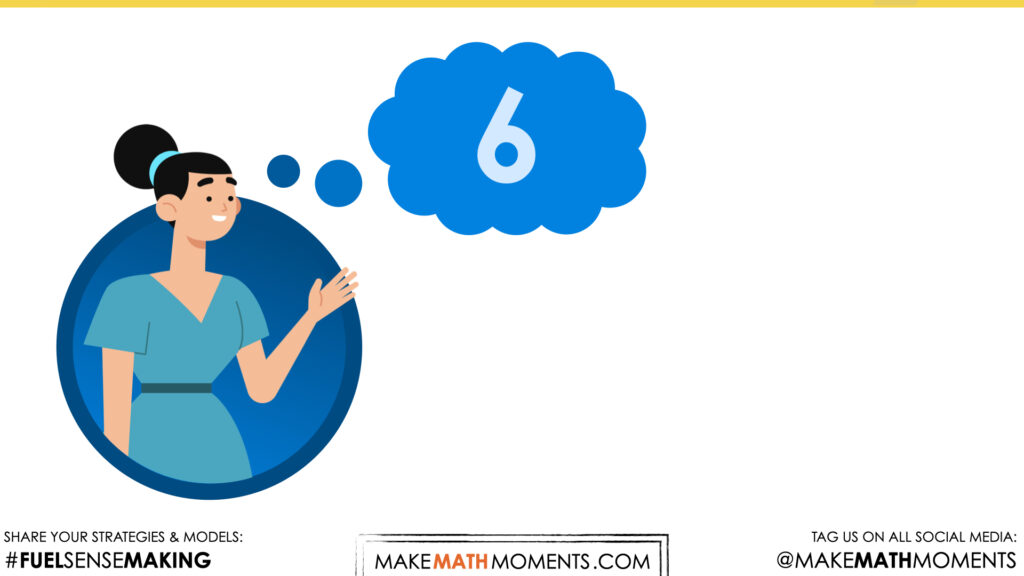This number talk starts with a visual to indicate that someone is thinking about a number.  Consider modelling the action of “putting one number in your head”, as the image implies. This may be done saying the number out while touching your head. The action can then be associated with the counting on strategy which will be explored in each of the number talks.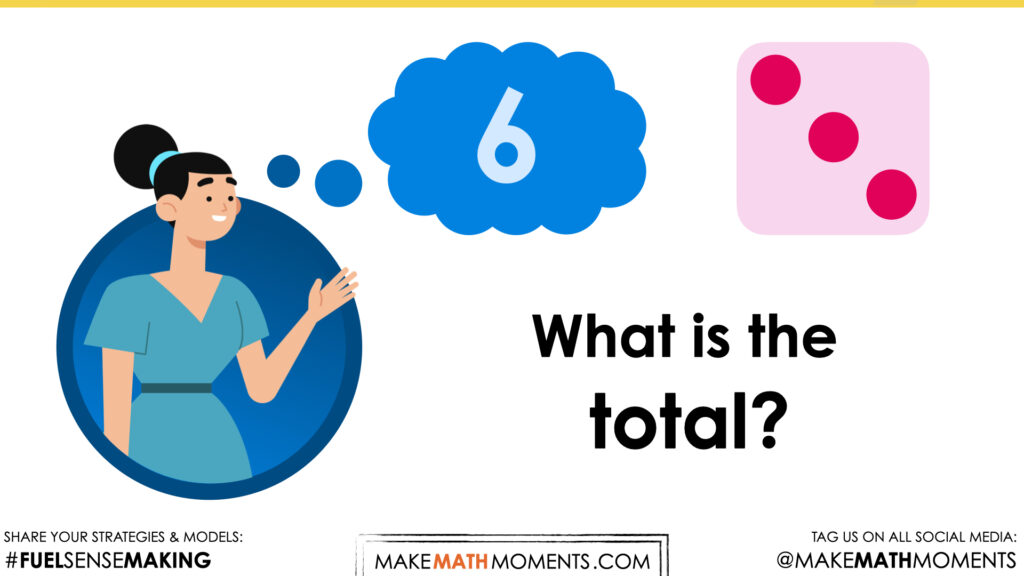The abstract number and the dots are purposefully chosen so that a student can hold one number in their head (as the picture implies) and then count on the remaining amount. This “putting one number in your head” action of counting on, demonstrates to students that they are going to remember that number therefore keeping it whole, then counting the other number. The dice pattern is also used to promote the discussion of subitizing.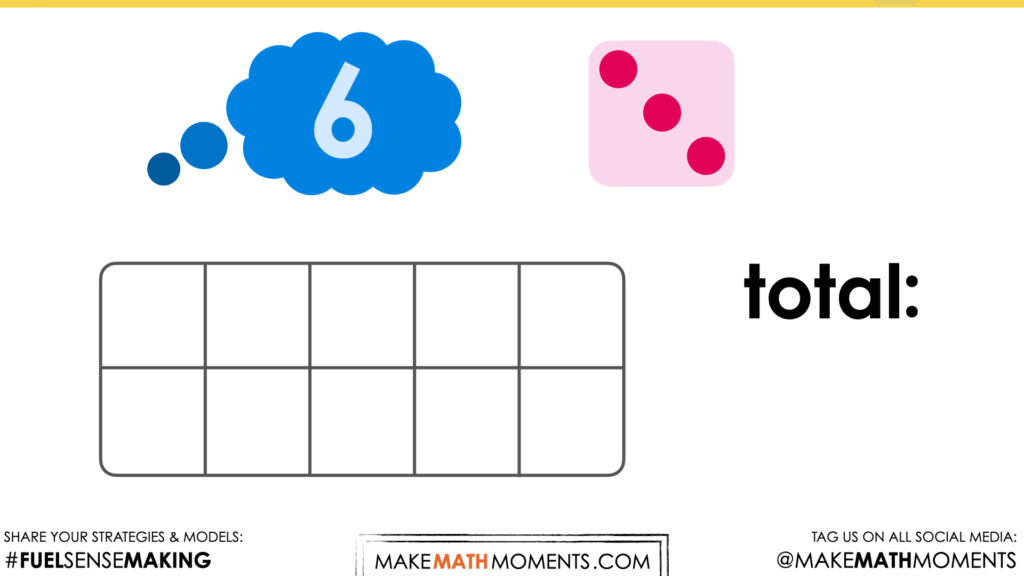A ten frame is introduced as a way to demonstrate how to solve this problem.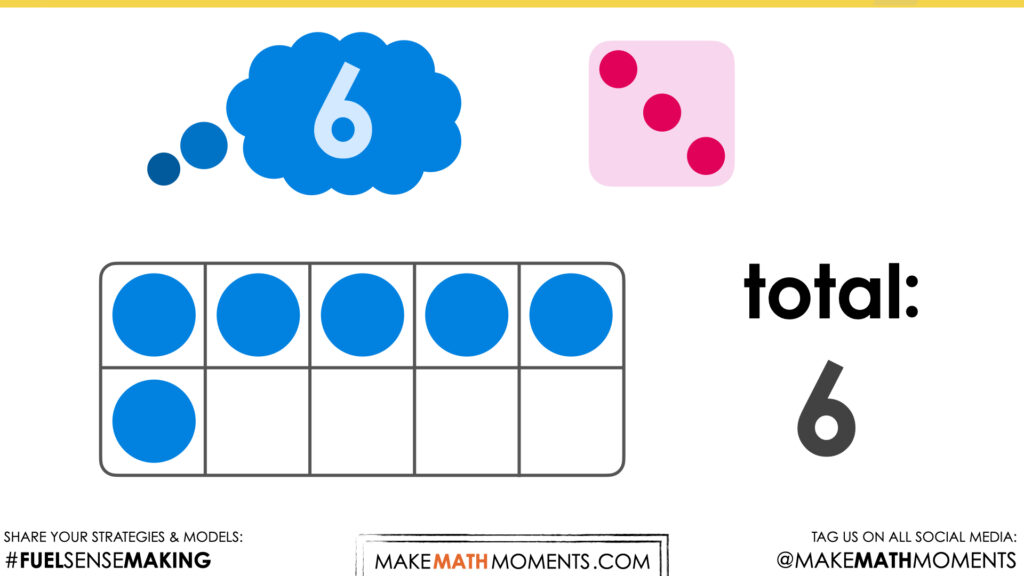The six dots are filled into the ten frames. This is another opportunity for students to see the amount that is being discussed.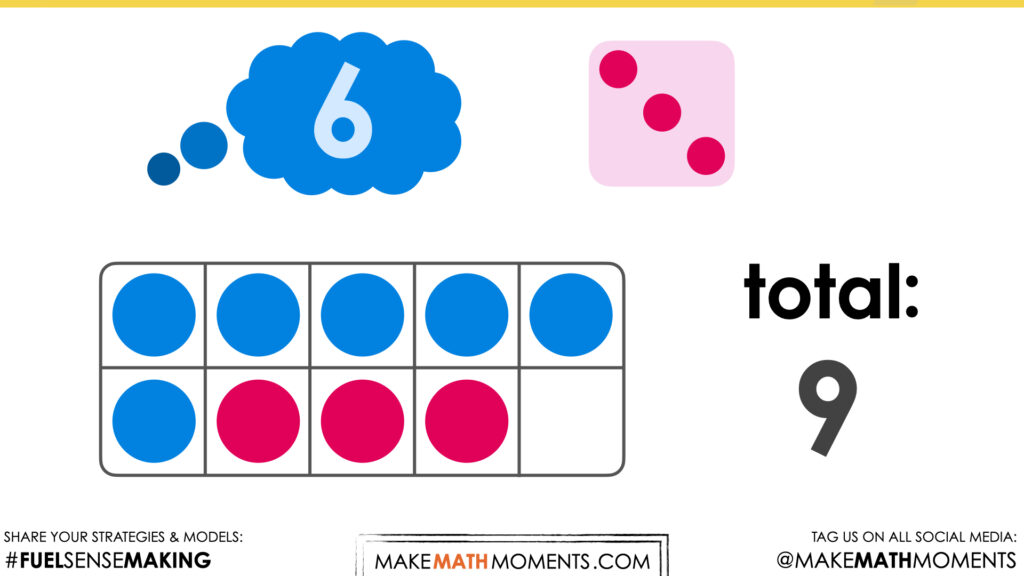The counting on strategy is demonstrated as the red dots are filled in to show the total amount of 9.

## Visual Math Talk Prompt #2

Play the video of the following visual number string prompt.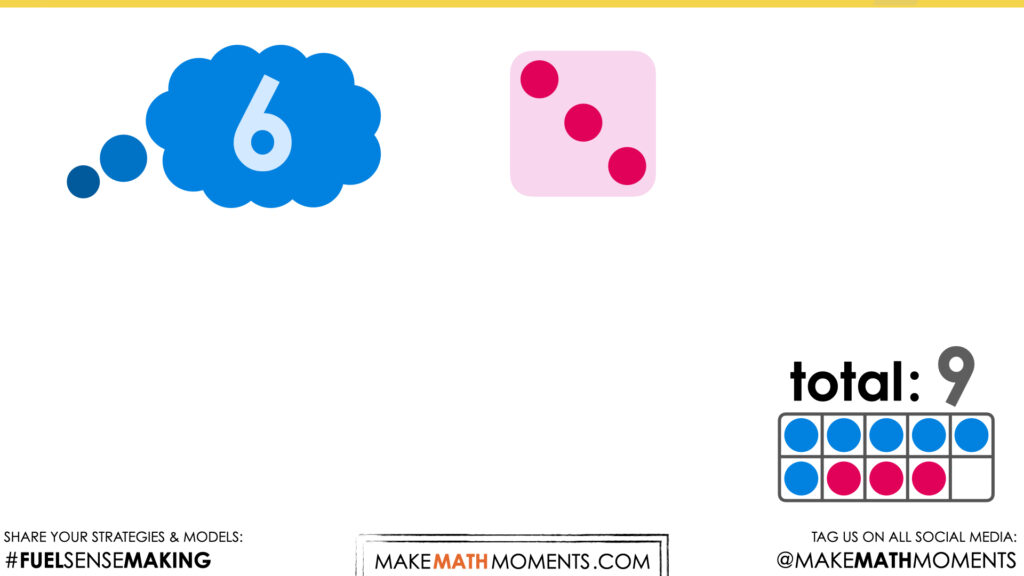This number talk continues from the result of number talk prompt 1.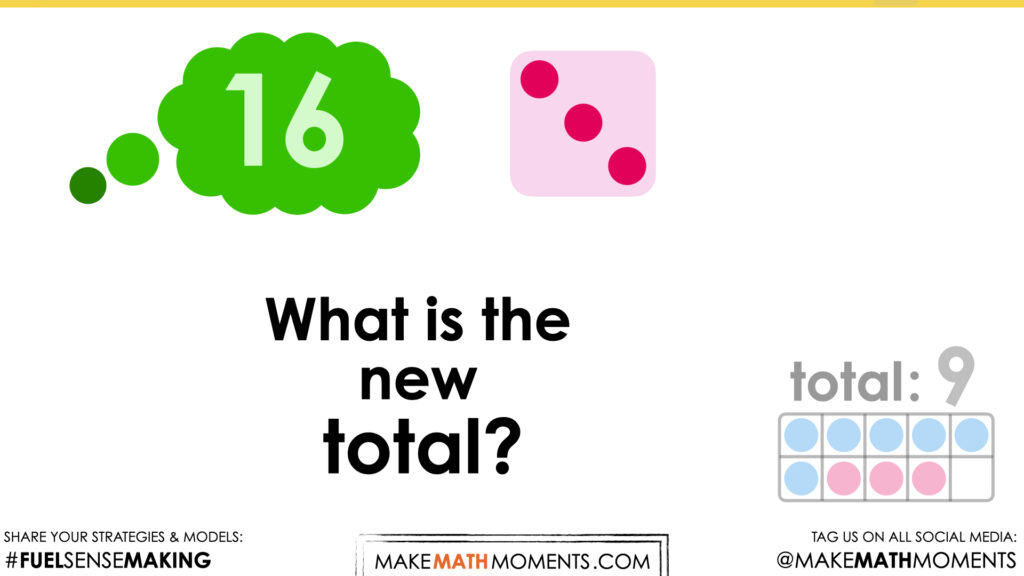This prompt is part of a “number string” as it builds on the previous prompt. The initial number of 6 has increased by ten to make a connection to the teen numbers. The numbers are within range to count on using fingers but are too high for a child to be able to count 3 times with manipulatives. In doing so, it creates a situation where a student may want to try the more sophisticated strategy of counting on to increase efficiency. Now that 16 does not need to be recounted, there is more room on fingers to count on.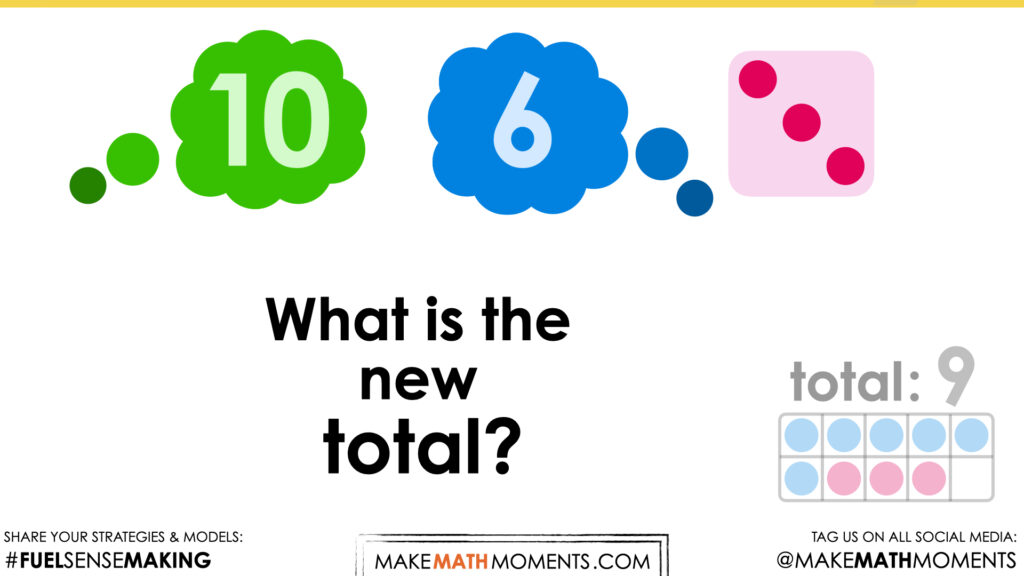The 16 has been decomposed into a 10 and 6 to show the connection to the first prompt. The answer to this prompt will be 10 more than the previous answer of 9.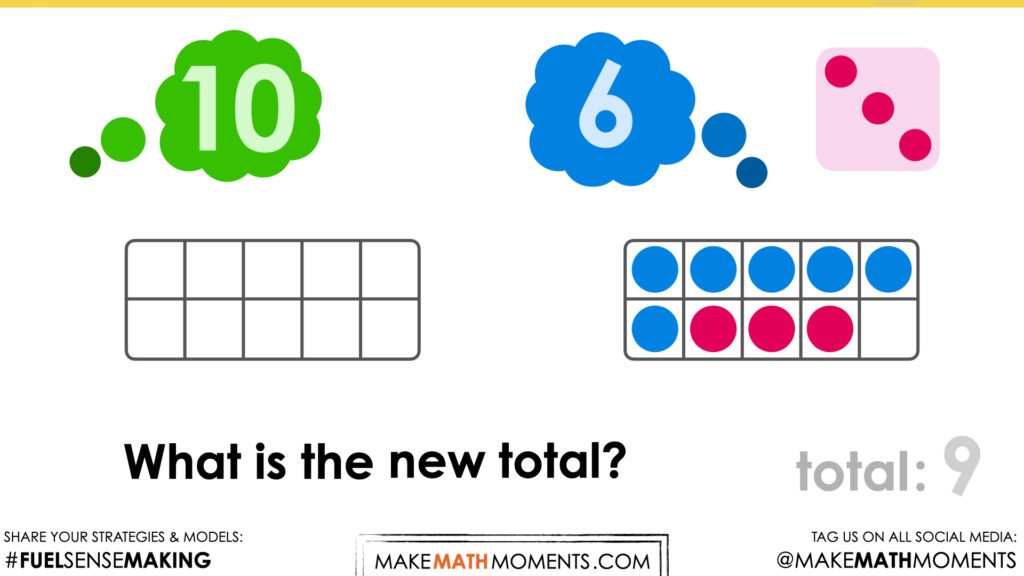The ten frame is brought back to again make the connection to the first prompt.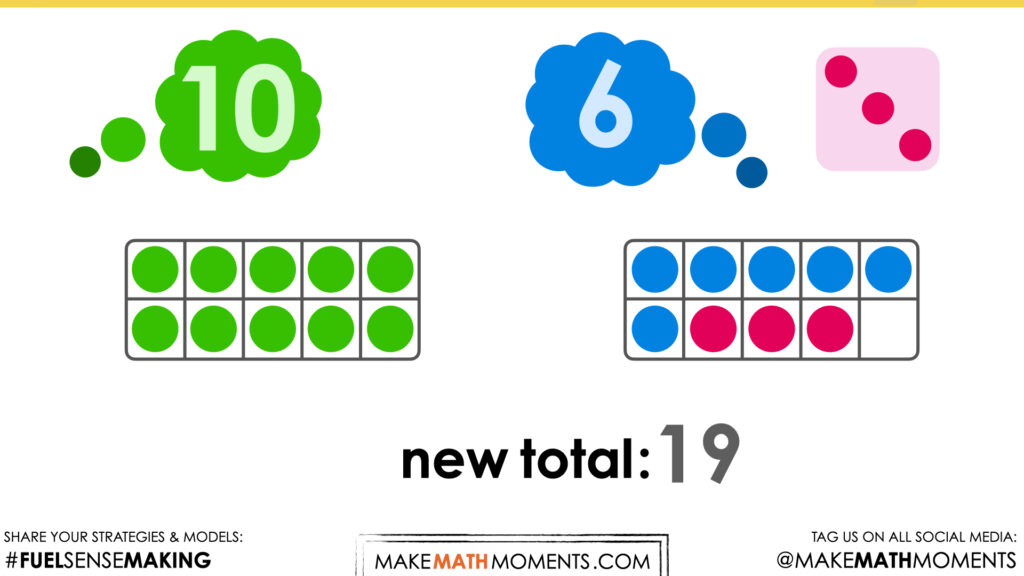The final result of the prompt is found by counting on 3 to 16, but also demonstrated here by using the result from the first prompt and counting on 10 more. At this stage, take time to acknowledge student strategies and particularly noting when students are using a counting on strategy.

## Visual Math Talk Prompt #3

Play the video of the following visual number string prompt.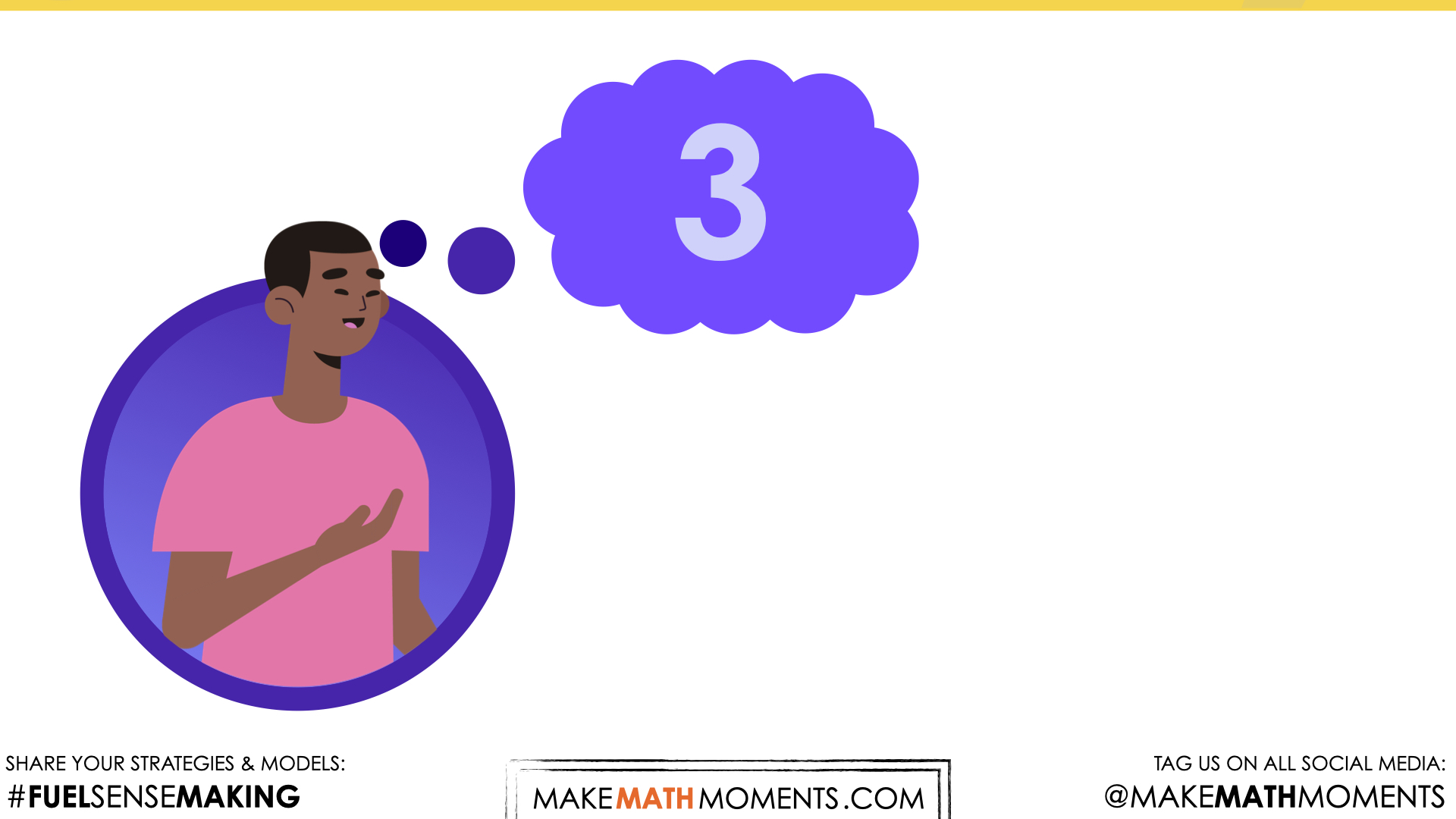Description of visual here: Similar to the first prompt, the image is set to promote the action of “putting a number in your head”. Again, consider modelling the action of putting the number 3 “in your head” so that number is left whole then the other number can be counted on.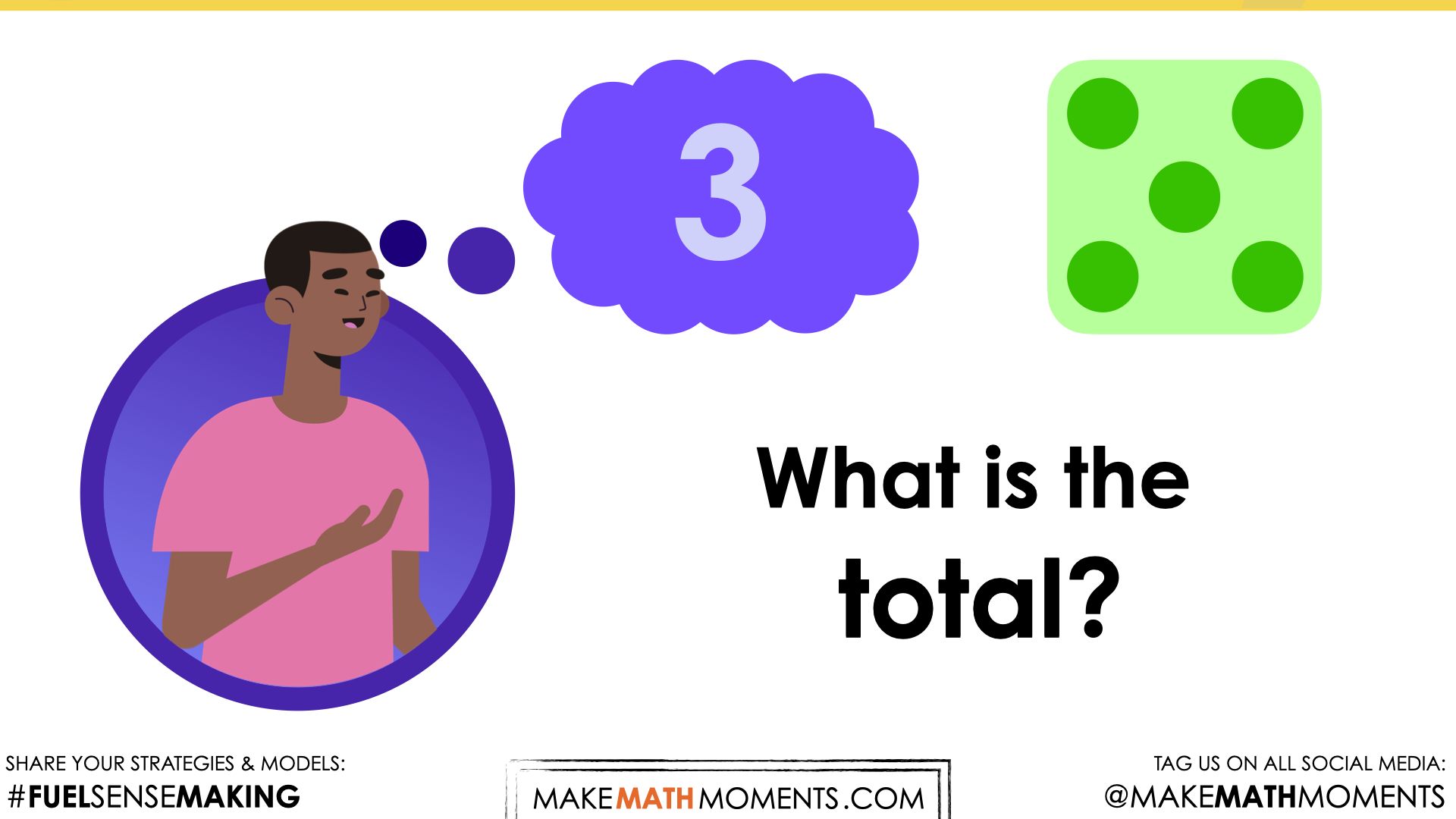Description of visual here: Now that the other number has been introduced, the students could be reminded that the number 3 is “in their head” and now there are 5 more to count on.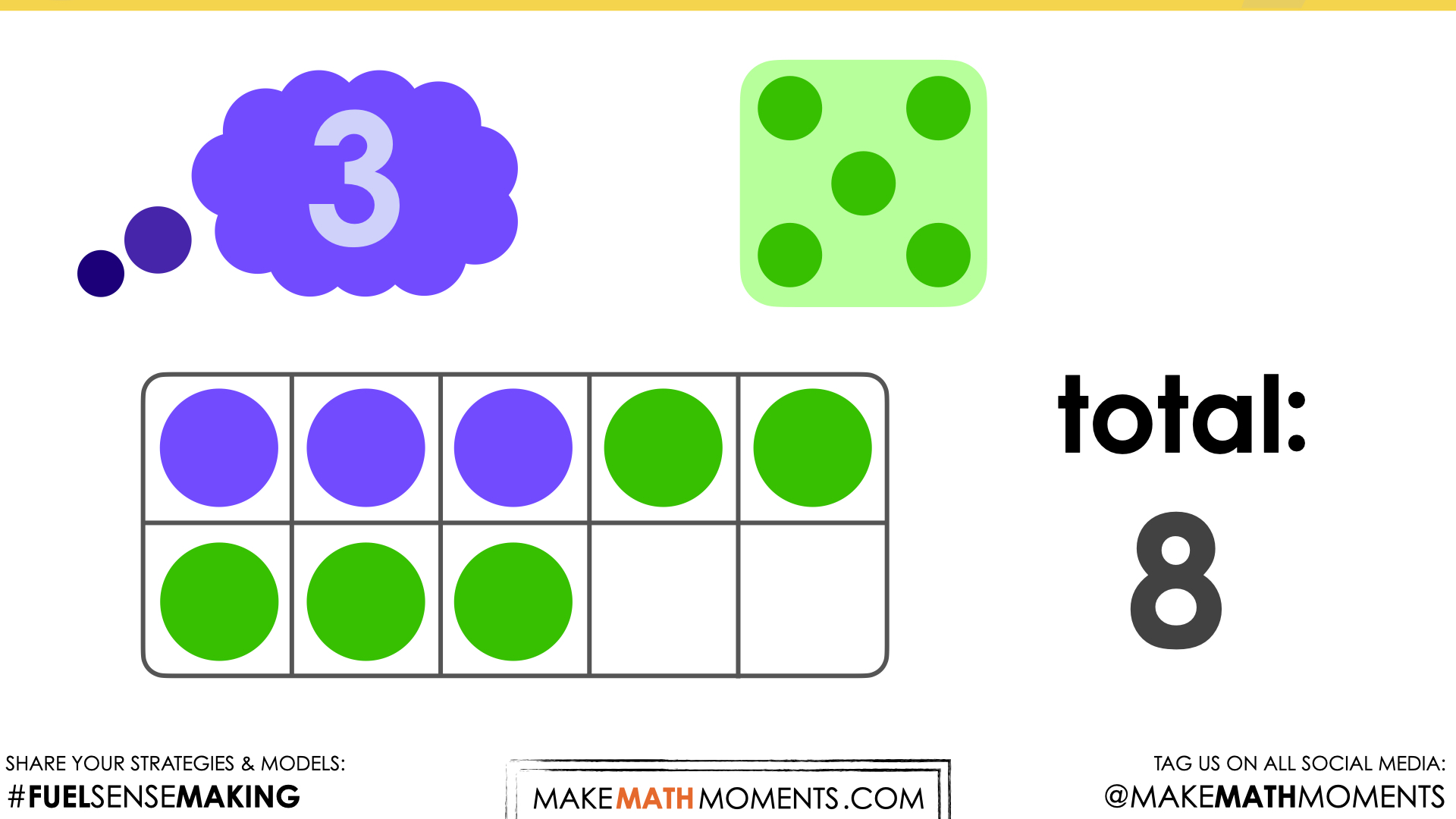Description of visual here: A ten frame is used to model the reveal of this prompt. It is another opportunity for students to create a visual of the numbers involved in the prompt as the counting on strategy is being used.

## Visual Math Talk Prompt #4

Play the video of the following visual number string prompt.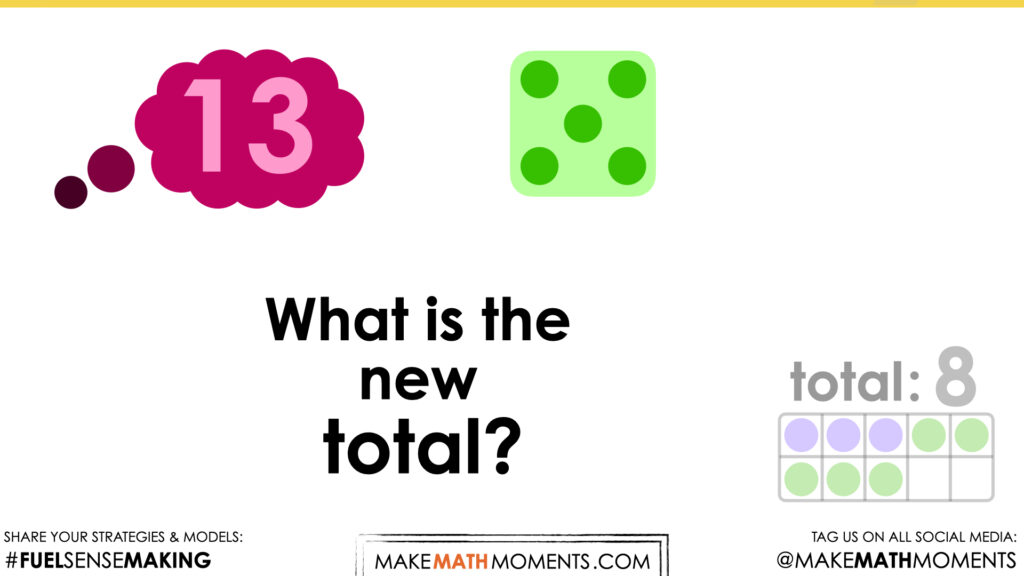This prompt follows the same number string pattern as prompt 1 and 2. The previous prompt was 3 + 5 and now 13 + 5.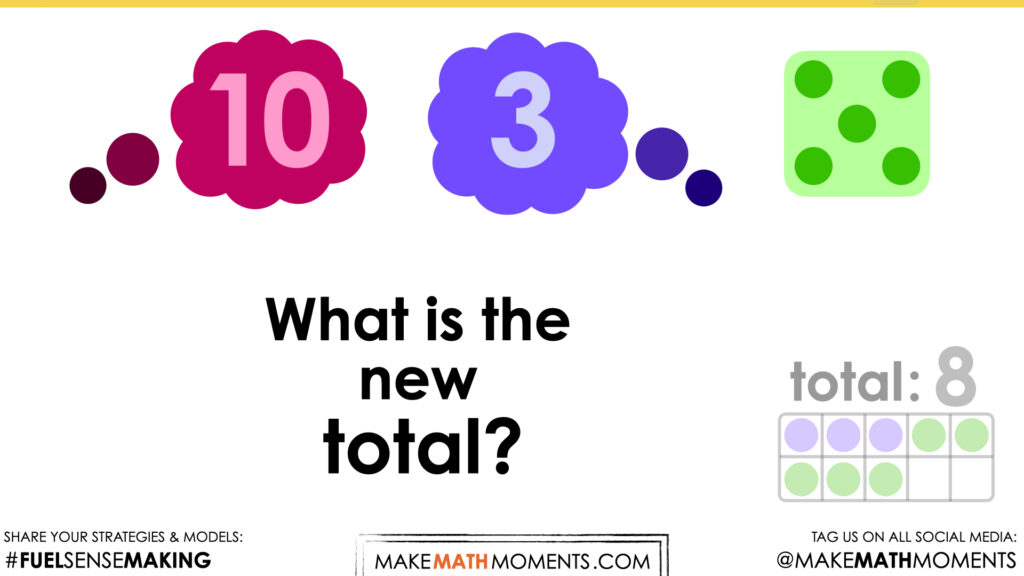Again, 13 is decomposed into 10 and 3 to make the connection to the previous prompt. Since it is the same structure as the prompt 1 and 2, students may make the connection that this answer is also just 10 more than 8.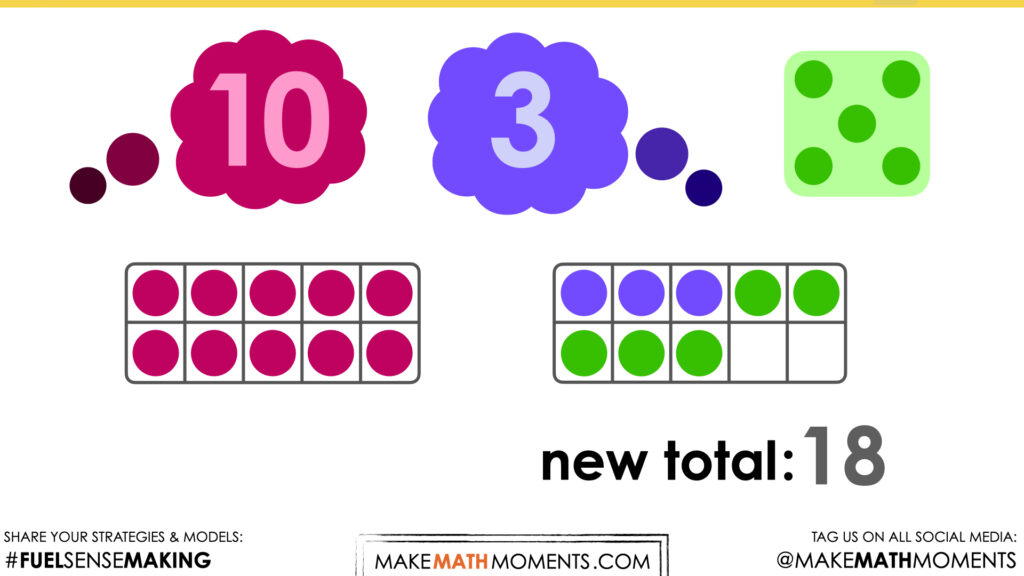To demonstrate the counting on strategy, students may not decompose the 13 into 10 and 3, so this final reveal may look different in classroom setting. This is just one way to demonstrate the counting on that could take place.

## Visual Math Talk Prompt #5

Play the video of the following visual number string prompt.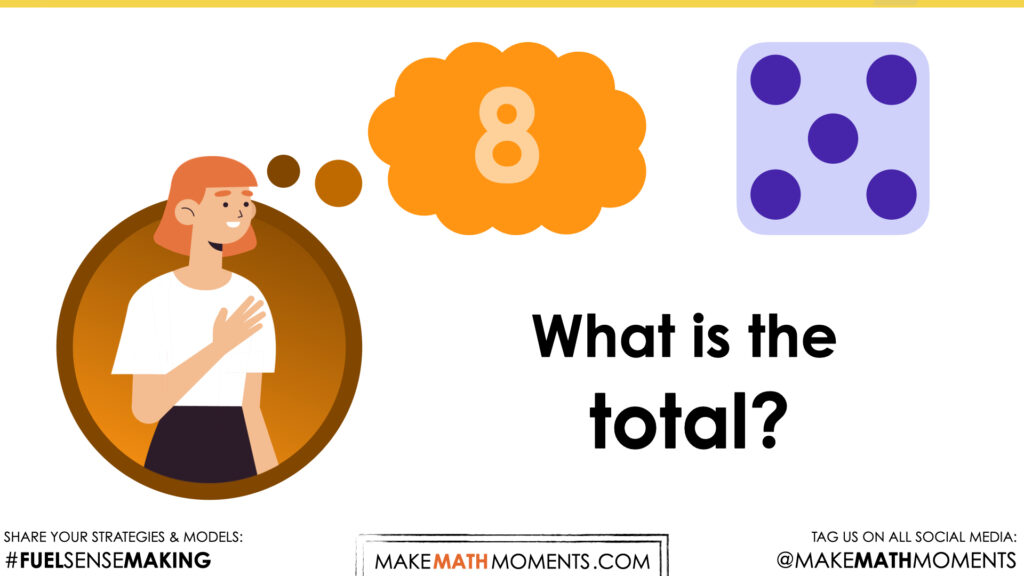This number talk is the start of a number string similar to the first four prompts. Consider demonstrating “putting 8 in their head” so 5 can be counted on.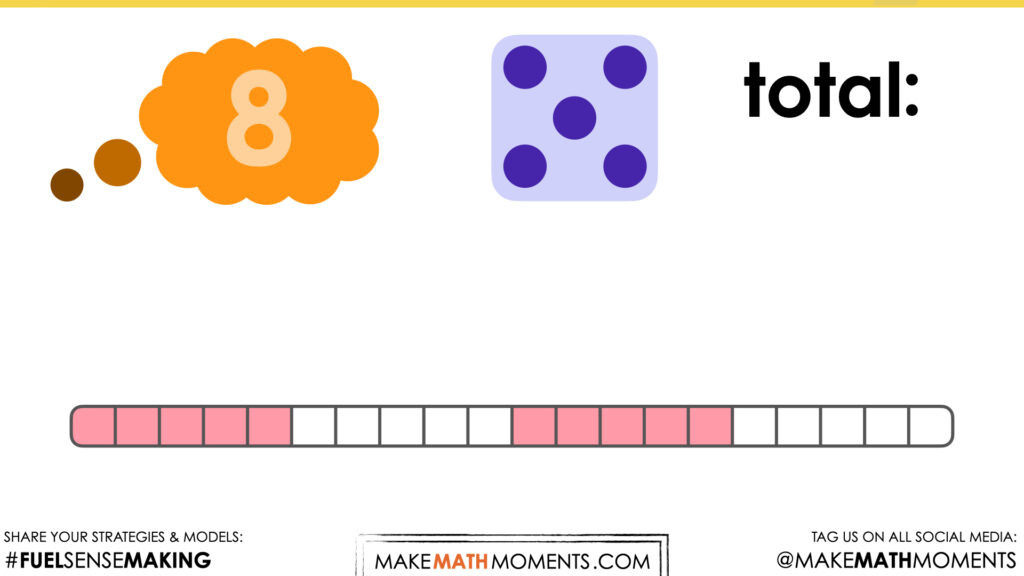Instead of a ten frame, a concrete number line is used in the reveal portion. By exposing students to different tools (ten frame and now a number line), it provides another opportunity for them to visual numbers.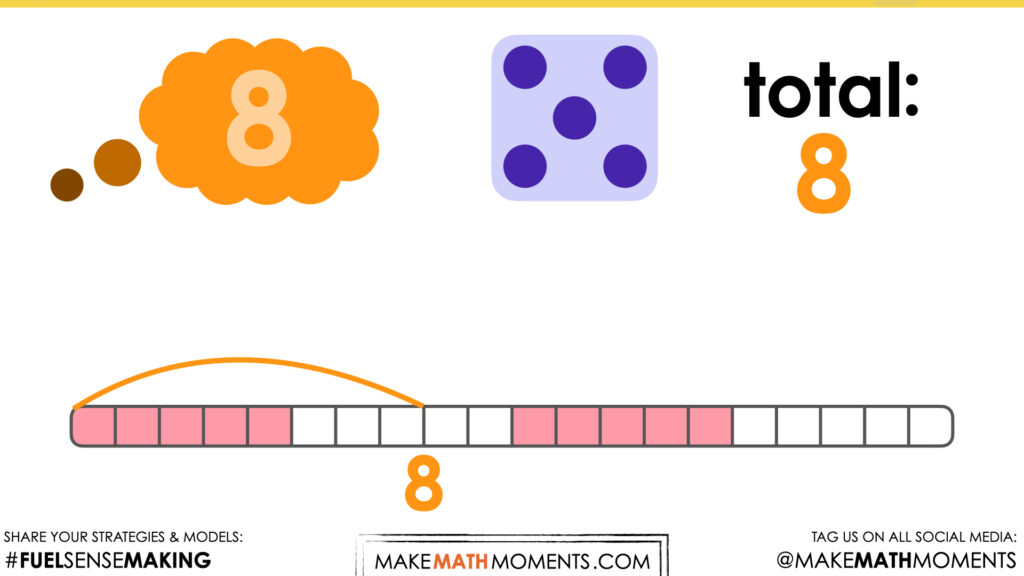The initial “jump” of 8 is purposefully done in one jump as a reminder that 8 is staying whole, it does not need to be “recounted”.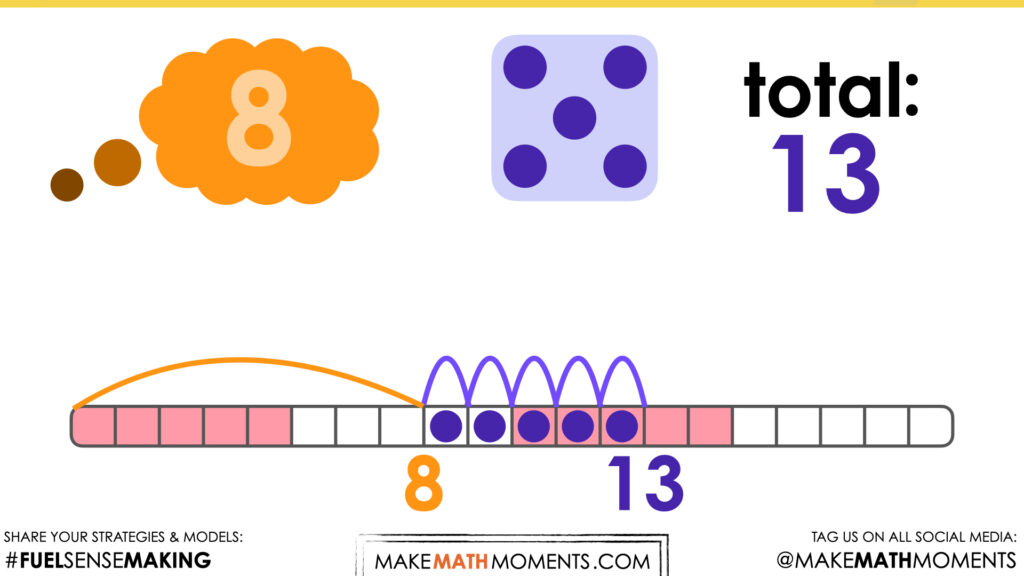The additional 5 is individually split into jumps of 1 at a time to show the counting on strategy in action on a number line.

## Visual Math Talk Prompt #6

Login/Join to access the full teacher guides, downloadable slide decks and printable handouts for this lesson and all problem based units.

## Visual Math Talk Prompt #7

Login/Join to access the full teacher guides, downloadable slide decks and printable handouts for this lesson and all problem based units.

## Visual Math Talk Prompt #8

Login/Join to access the full teacher guides, downloadable slide decks and printable handouts for this lesson and all problem based units.

## Visual Math Talk Prompt #9

Login/Join to access the full teacher guides, downloadable slide decks and printable handouts for this lesson and all problem based units.

## Visual Math Talk Prompt #10

Login/Join to access the full teacher guides, downloadable slide decks and printable handouts for this lesson and all problem based units.

## Working With Circles: Circumference

Login/Join to access the full teacher guides, downloadable slide decks and printable handouts for this lesson and all problem based units.

## Practice Problems: Game – Flip a Head, Roll a Hand

Login/Join to access the entire Teacher Guide, downloadable slide decks and printable handouts for this lesson and all problem based units.

## Purposeful Practice Station #2: Game – Spinny Roll

Login/Join to access the entire Teacher Guide, downloadable slide decks and printable handouts for this lesson and all problem based units.

## Purposeful Practice: Small Group Activities

Login/Join to access the entire Teacher Guide, downloadable slide decks and printable handouts for this lesson and all problem based units.

Login/Join to access the entire Teacher Guide, downloadable slide decks and printable handouts for this lesson and all problem based units.

## Educator Discussion Area

Login/Join to access the entire Teacher Guide, downloadable slide decks and printable handouts for this lesson and all problem based units.

## Explore Our 60+ Problem Based Units

This Make Math Moments Lesson was designed to spark curiosity for a multi-day unit of study with built in purposeful practice, number talks and extensions to elicit and emerge strategies and mathematical models.

Dig into our other units of study and view by concept continuum, grade or topic!